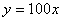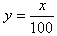Introduction

An inverse function is a function that undoes another function, much like addition undoes subtraction. Inverse functions use inverse operations to turn inputs into outputs and vice versa.

• If a function answers the question: "Alice worked this long; how much money has she made?" Then its inverse answers the question: "Alice made this much money; how long did she work?"
• If a function answers the question: "How many hours of music fit on 12 CDs?" Then its inverse answers the question: "How many CDs do you need for 3 hours of music?"

If a function is modeled by the rule,, then the inverse function is modeled by. Since multiplication and division are inverse operations, they will create inverse functions.

The function, f -1(x), is defined as the inverse function of f(x) if it consistently reverses the process of f(x). That is, if f(x) turns a into b, then f -1(x) must turn b into a. f -1(x) is not an exponent. It is meant to be an inverse function, not a reciprocal.

The inverse has all the same points as the original function, except that the x and y-coordinates have been reversed. If the point (3,2) is a point in the original function then the point (2,3) will be a point of the inverse function.

It is possible to find the inverse of a set of points, a table, a graph or an equation. The set of points and the table work the same way. All three methods for finding inverses of functions will be discussed here.

Listen